• （3）判断文法是否为SLR文法 （4）输入字符串，按照SLR分析法判断该字符串的语法是否正确，并给出判断过程 代码的思路： 将文法中的每一个句子的左部和右部进行分离。其中，右部的每一个终结符和非终结符也是...
代码功能：

（1）计算非终结符的FIRST和FOLLOW集

（2）构造SLR分析表

（3）判断该文法是否为SLR文法

（4）输入字符串，按照SLR分析法判断该字符串的语法是否正确，并给出判断过程

代码的思路：

将文法中的每一个句子的左部和右部进行分离。其中，右部的每一个终结符和非终结符也是单独保存的。
将左部和右部分离后的文法中的终结符和非终结符识别出来。一般左部都是非终结符，那么右部除去非终结符就是终结符了。
计算FIRST集。设置一个变量_ALTERED_，在每次有新的终结符添加到FIRST集中的时候，就将_ALTERED_置位true。计算FIRST集的方法如下：如果产生式右部是终结符a，且这个终结符a不在FIRST集中，则添加。如果是非终结符A，则将A的FIRST集中的所有自己没有的终结符添加到自己的FIRST集中。如果A的终结符中包含ε，则继续判断下一个。
计算FOLLOW集。设置一个变量_ALTERED_，在每次有新的终结符添加到FOLLOW集中的时候，就将_ALTERED_置位true。计算FOLLOW集的方法如下：1、遍历文法中的所有产生式，所有出现在非终结符右边的终结符加入到相应follow集中，如果非终结符右边没有东西，则把$加入。 2、遍历文法中的所有产生式，若产生式最右边是非终结符，把左部非终结符的follow集的所有元素都添加到对应非终结符中。3、将$加入到第一个非终结符的follow集中。
根据每一条产生式，生成项目集规范族。程序设计的是对项目集规范族中的每一个项目集中的每一条项目都进行分析，产生了新的项目集就添加到项目集规范族中。
根据项目集规范族和FOLLOW集生成SLR分析表。根据·的位置判断是否将其添加到SLR分析表中，很容易。如果·后面的是终结符，将转移动作s添加到SLR分析表中的ACTION部分；如果·后面的是终结符，将转移添加到SLR分析表的GOTO部分；如果·在项目的末尾，将归约动作r添加到SLR分析表的ACTION部分。
设计词法分析器，用于分析输入的程序。
根据SLR分析表和词法分析的结果进行词法分析。
程序代码：

GitHub上的项目：https://github.com/z1178902213/SLRAnalyser-

程序运行：

文法：

E->E+T
E->E-T
E->T
T->T*K
T->T/K
T->K
K->K%F
K->K^F
K->F
F->(E)
F->id
F->num

测试程序：

a+xyz*10+(c/d)+(xyz%a)+(d^5)

运行结果：

终结符：[+, -, *, /, %, ^, (, ), id, num]
非终结符：[E, T, K, F]
文法如下所示：
(1)E->E+T
(2)E->E-T
(3)E->T
(4)T->T*K
(5)T->T/K
(6)T->K
(7)K->K%F
(8)K->K^F
(9)K->F
(10)F->(E)
(11)F->id
(12)F->num

文法的First集为：
First(E) = { ( id num }
First(T) = { ( id num }
First(K) = { ( id num }
First(F) = { ( id num }

文法的Follow集为：
Follow(E) = { + - ) $} Follow(T) = {$ * / + - ) }
Follow(K) = { $% ^ * / + - ) } Follow(F) = {$ % ^ * / + - ) }

该文法的项目集规范族如下所示：
I0：
START->·E
E->·E+T
E->·E-T
E->·T
T->·T*K
T->·T/K
T->·K
K->·K%F
K->·K^F
K->·F
F->·(E)
F->·id
F->·num

I1：
START->E·
E->E·+T
E->E·-T

I2：
E->T·
T->T·*K
T->T·/K

I3：
T->K·
K->K·%F
K->K·^F

I4：
K->F·

I5：
F->(·E)
E->·E+T
E->·E-T
E->·T
T->·T*K
T->·T/K
T->·K
K->·K%F
K->·K^F
K->·F
F->·(E)
F->·id
F->·num

I6：
F->id·

I7：
F->num·

I8：
E->E+·T
T->·T*K
T->·T/K
T->·K
K->·K%F
K->·K^F
K->·F
F->·(E)
F->·id
F->·num

I9：
E->E-·T
T->·T*K
T->·T/K
T->·K
K->·K%F
K->·K^F
K->·F
F->·(E)
F->·id
F->·num

I10：
T->T*·K
K->·K%F
K->·K^F
K->·F
F->·(E)
F->·id
F->·num

I11：
T->T/·K
K->·K%F
K->·K^F
K->·F
F->·(E)
F->·id
F->·num

I12：
K->K%·F
F->·(E)
F->·id
F->·num

I13：
K->K^·F
F->·(E)
F->·id
F->·num

I14：
F->(E·)
E->E·+T
E->E·-T

I15：
E->E+T·
T->T·*K
T->T·/K

I16：
E->E-T·
T->T·*K
T->T·/K

I17：
T->T*K·
K->K·%F
K->K·^F

I18：
T->T/K·
K->K·%F
K->K·^F

I19：
K->K%F·

I20：
K->K^F·

I21：
F->(E)·

SLR分析表：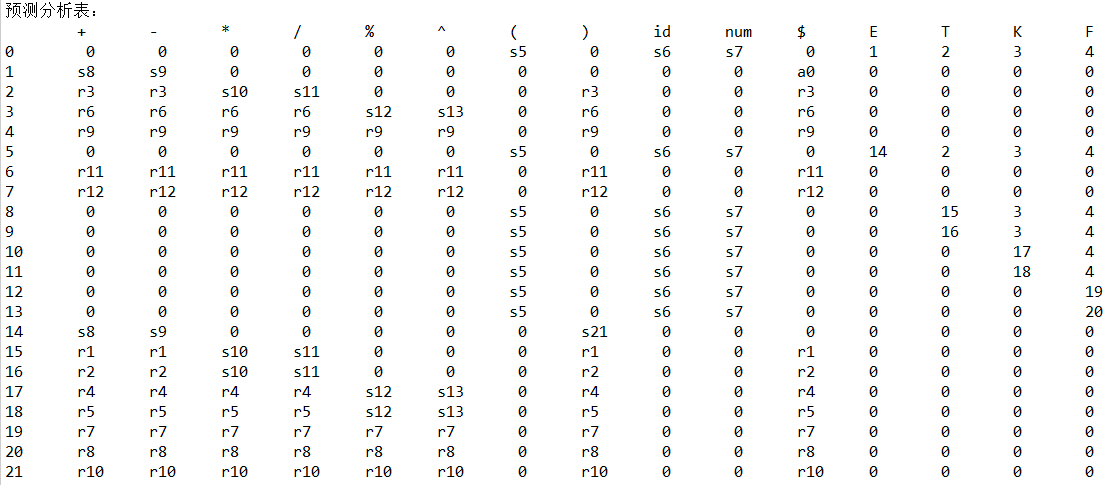栈                                输入        动作
0                                         a+xyz*10+(c/d)+(xyz%a)+(d^5)$移进 0id6 +xyz*10+(c/d)+(xyz%a)+(d^5)$        按F->[id]归约
0F4                                       +xyz*10+(c/d)+(xyz%a)+(d^5)$按K->[F]归约 0K3 +xyz*10+(c/d)+(xyz%a)+(d^5)$        按T->[K]归约
0T2                                       +xyz*10+(c/d)+(xyz%a)+(d^5)$按E->[T]归约 0E1 +xyz*10+(c/d)+(xyz%a)+(d^5)$        移进
0E1+8                                     xyz*10+(c/d)+(xyz%a)+(d^5)$移进 0E1+8id6 *10+(c/d)+(xyz%a)+(d^5)$        按F->[id]归约
0E1+8F4                                    *10+(c/d)+(xyz%a)+(d^5)$按K->[F]归约 0E1+8K3 *10+(c/d)+(xyz%a)+(d^5)$        按T->[K]归约
0E1+8T15                                   *10+(c/d)+(xyz%a)+(d^5)$移进 0E1+8T15*10 10+(c/d)+(xyz%a)+(d^5)$        移进
0E1+8T15*10num7                               +(c/d)+(xyz%a)+(d^5)$按F->[num]归约 0E1+8T15*10F4 +(c/d)+(xyz%a)+(d^5)$        按K->[F]归约
0E1+8T15*10K17                                +(c/d)+(xyz%a)+(d^5)$按T->[T, *, K]归约 0E1+8T15 +(c/d)+(xyz%a)+(d^5)$        按E->[E, +, T]归约
0E1                                           +(c/d)+(xyz%a)+(d^5)$移进 0E1+8 (c/d)+(xyz%a)+(d^5)$        移进
0E1+8(5                                         c/d)+(xyz%a)+(d^5)$移进 0E1+8(5id6 /d)+(xyz%a)+(d^5)$        按F->[id]归约
0E1+8(5F4                                        /d)+(xyz%a)+(d^5)$按K->[F]归约 0E1+8(5K3 /d)+(xyz%a)+(d^5)$        按T->[K]归约
0E1+8(5T2                                        /d)+(xyz%a)+(d^5)$移进 0E1+8(5T2/11 d)+(xyz%a)+(d^5)$        移进
0E1+8(5T2/11id6                                    )+(xyz%a)+(d^5)$按F->[id]归约 0E1+8(5T2/11F4 )+(xyz%a)+(d^5)$        按K->[F]归约
0E1+8(5T2/11K18                                    )+(xyz%a)+(d^5)$按T->[T, /, K]归约 0E1+8(5T2 )+(xyz%a)+(d^5)$        按E->[T]归约
0E1+8(5E14                                         )+(xyz%a)+(d^5)$移进 0E1+8(5E14)21 +(xyz%a)+(d^5)$        按F->[(, E, )]归约
0E1+8F4                                             +(xyz%a)+(d^5)$按K->[F]归约 0E1+8K3 +(xyz%a)+(d^5)$        按T->[K]归约
0E1+8T15                                            +(xyz%a)+(d^5)$按E->[E, +, T]归约 0E1 +(xyz%a)+(d^5)$        移进
0E1+8                                                (xyz%a)+(d^5)$移进 0E1+8(5 xyz%a)+(d^5)$        移进
0E1+8(5id6                                               %a)+(d^5)$按F->[id]归约 0E1+8(5F4 %a)+(d^5)$        按K->[F]归约
0E1+8(5K3                                                %a)+(d^5)$移进 0E1+8(5K3%12 a)+(d^5)$        移进
0E1+8(5K3%12id6                                            )+(d^5)$按F->[id]归约 0E1+8(5K3%12F19 )+(d^5)$        按K->[K, %, F]归约
0E1+8(5K3                                                  )+(d^5)$按T->[K]归约 0E1+8(5T2 )+(d^5)$        按E->[T]归约
0E1+8(5E14                                                 )+(d^5)$移进 0E1+8(5E14)21 +(d^5)$        按F->[(, E, )]归约
0E1+8F4                                                     +(d^5)$按K->[F]归约 0E1+8K3 +(d^5)$        按T->[K]归约
0E1+8T15                                                    +(d^5)$按E->[E, +, T]归约 0E1 +(d^5)$        移进
0E1+8                                                        (d^5)$移进 0E1+8(5 d^5)$        移进
0E1+8(5id6                                                     ^5)$按F->[id]归约 0E1+8(5F4 ^5)$        按K->[F]归约
0E1+8(5K3                                                      ^5)$移进 0E1+8(5K3^13 5)$        移进
0E1+8(5K3^13num7                                                 )$按F->[num]归约 0E1+8(5K3^13F20 )$        按K->[K, ^, F]归约
0E1+8(5K3                                                        )$按T->[K]归约 0E1+8(5T2 )$        按E->[T]归约
0E1+8(5E14                                                       )$移进 0E1+8(5E14)21$        按F->[(, E, )]归约
0E1+8F4                                                           $按K->[F]归约 0E1+8K3$        按T->[K]归约
0E1+8T15                                                          $按E->[E, +, T]归约 0E1$        接受
程序正确


展开全文JAVA 编译原理
• 思路：依次判断是否为LL(1)文法SLR文法即可 证明： 　(1)首先该文法无左递归存在,没有公共左因子. 　其次:对于S→AaAb|BbBa FIRST(AaAb)={a} FIRST(BbBa)={b} 　FIRST(AaAb)∩FIRST(BbBa)=Φ 　所以该文法是LL(1)...
思路：依次判断是否为LL(1)文法和SLR文法即可
证明：
(1)首先该文法无左递归存在,没有公共左因子.
其次:对于S→AaAb|BbBa FIRST(AaAb)={a} FIRST(BbBa)={b}
FIRST(AaAb)∩FIRST(BbBa)=Φ
所以该文法是LL(1)文法.
(2)证明该文法不是SLR的.
文法的LR(0)项目集规范族为:
I0={S’→.S S→.AaAb S→.BbBa A→. B→.}
I1={ S’→ S. }
I2={ S→A.aAb }
I3={ S→B.bBa }
I4={ S→Aa.Ab A→. }
I5={ S→Bb.Ba B→. }
I6={ S→AaA.b }
I7={ S→BbB.a }
I8={ S→AaAb. }
I9={ S→BbBa. }
考察I0:
FOLLOW(A)={a,b} FOLLOW(B)={a,b} FOLLOW(A)∩FOLLOW(B)= {a,b}
产生规约-规约冲突.
所以该文法不是SLR(1)文法.


展开全文• 该程序能够根据给定的文法判断它是否为LR0，SLR1，LR1，LALR1文法； 打印项目集，分析表，Go函数； 若文法属于LR1，将进行LALR1文法判断，若属于LALR1文法，将继续打印LALR1文法的项目集，分析表和Go函数。
• 1、算符优先分析法（需要先来判断文法是否为算符优先文法） 2、LR（0）分析法 3、SLR1）分析法 该程序的功能为，给定输入，程序按照先后顺序将使用的产生式输出。 如，输入25.6 * 14.5 + 2（首先经过词法分析，将...
• 1． 对输入的文法进行判断，是否为相应SLR文法，若不是提示重新输入文法。 2． 输出相应的项目集规范簇 3． 输出相应的LR分析表。 4． 输入一个句子，输出其分析过程（移进，归约，接受）
• 推论3.2二、LR(0)和SLR(1)1. 一、LL(1) 1.意思 第一个L代表从左到右扫描输入序列，第二个L表示产生最左推导，1表示在确定分析器的每一步动作时向前看一个终结符。 2.判断 当且仅当为它构造的预测分析表中不含多重...
文章目录一、LL(1)1.意思2.判断3.推论3.2二、LR(0)和SLR(1)1.判断

【编译原理博客列表】》》》》》》

一、LL(1)
1.意思
第一个L代表从左到右扫描输入序列，第二个L表示产生最左推导，1表示在确定分析器的每一步动作时向前看一个终结符。
2.判断

有左递归和左因子的文法不是LL(1)文法。
当且仅当为它构造的预测分析表中不含多重定义的条目（即每个非终结符的FIRST集和FOLLOW集没有交集），才是LL(1)文法。

Created with Raphaël 2.2.0文法有左递归和左因子？不是LL(1)文法每个非终结符的FIRST集和FOLLOW集有交集？是LL(1)文法yesnoyesno

例：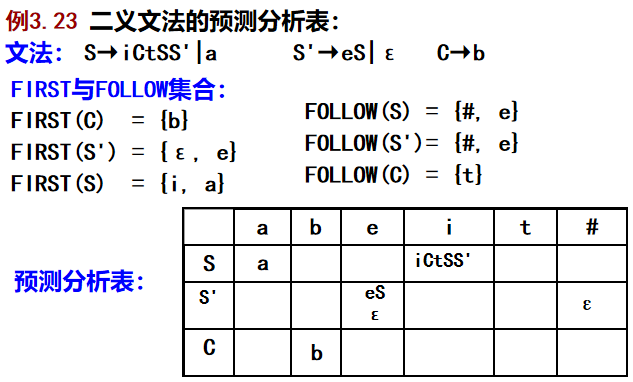有交集不是。
3.推论3.2
G是LL(1)的，当且仅当G的任何两个产生式A→α|β满足:

对任何终结符a，α和β不能同时推导出以a开始的串；
α和β最多有一个可以推导出ε；
若$β=*>ε$，则α不能导出以FOLLOW(A)中终结符开始的任何串。

二、LR(0)和SLR(1)
1.判断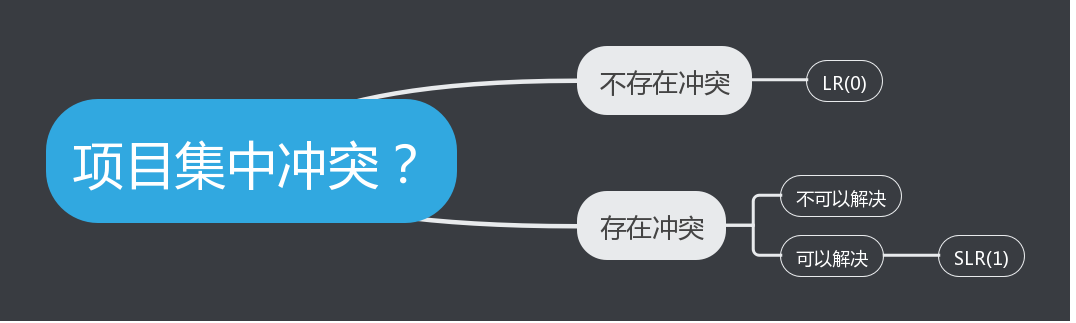二义文法不是SLR(1)文法


展开全文• 1.E->T{+T|-T} 2.T->F{*F|/F} 3.F->(E)|a|b|c 我采用的是递归下降分析法，它的基本思想是，对文法中的每个非终结符编写一个函数(或子程序)，每个函数（或子程序）的功能是识别由该非终结符所表示的语法成分。则...
• 实现了自制的C--语言的一遍扫描编译，包括词法分析，LR（1）语法分析，属性文法+中间代码生成，MIPS编译生成编译脚本由Python实现，兼容python2.7与3.7，图形界面由WPF实现，使用了IronPython进行脚本执行 ...
• LL（1文法 属于自上而下的分析方法。 也就是说，同一个非终结符的多种递推方式中，首字母一定不同。这样就可以只用根据一个首字母就可以判断出是哪一个递推式子。 文法名字由来 第一个L代表从左边开始扫描； 第二...
LL（1）文法
属于自上而下的分析方法。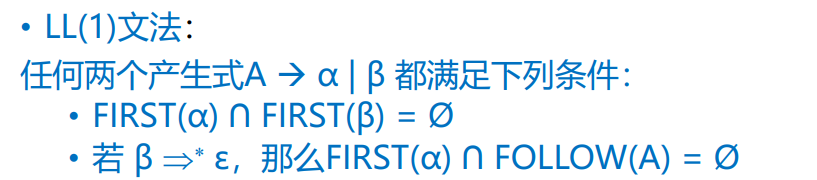也就是说，同一个非终结符的多种递推方式中，首字母一定不同。这样就可以只用根据一个首字母就可以判断出是哪一个递推式子。
文法名字由来
第一个L代表从左边开始扫描；
第二个L表示产生最左推导
数字1表示每一步推导式只需要向后看一个符号就可以
LL(1)文法的明显性质

没有公共左因子（如果有，那么无法只读一个字符就判断如何递归）
不是二义的（每个读入的字符都能确定具体含义）
不含有左递归（不包含回溯）

LR（SLR基于LR（0），LR（1）存在缺点，LALR进行折衷）
属于自下而上的分析方法。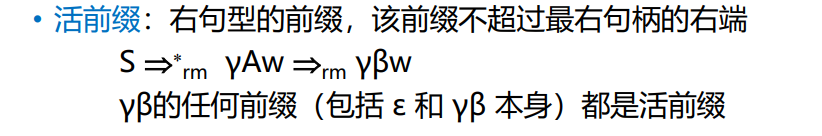栈中文法符号必定是构成一个活前缀
分析表中建立转移函数，本质上转移函数就是识别活前缀的DFA（有限状态转换自动机）
栈顶的状态符号包含了确定句柄所需要的一切信息；因为根据栈顶的状态符号和分析表就可以唯一地确定下一步要做什么。

缺点：
手工构造分析表的工作量太大了。
现在一般用机器生成分析表，可以找到相关开源软件。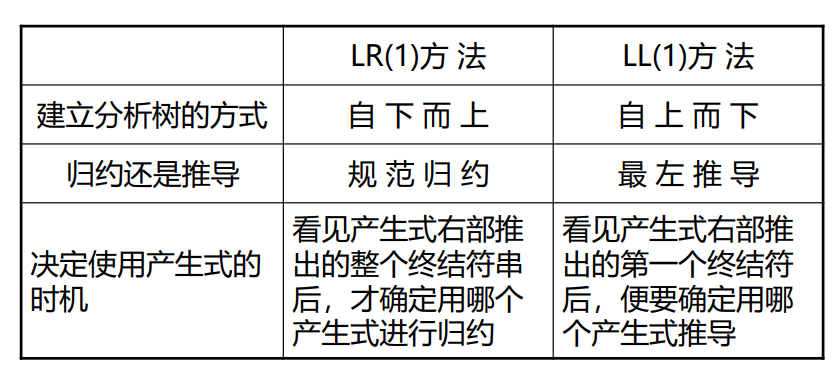SLR（1）分析表
简单的LR方法，简称SLR；它功能最弱但是最容易实现。
构造脱胎于LR（0）分析表
根据期待的不同后继来决定归约方式。
构造SLR分析表的两大步骤

从文法构造识别活前缀的DFA
从上述活前缀的DFA构造分析表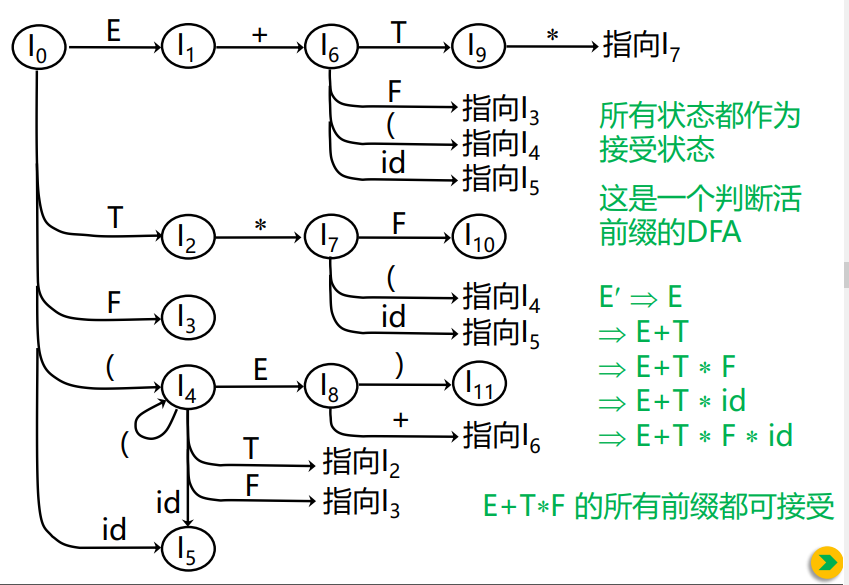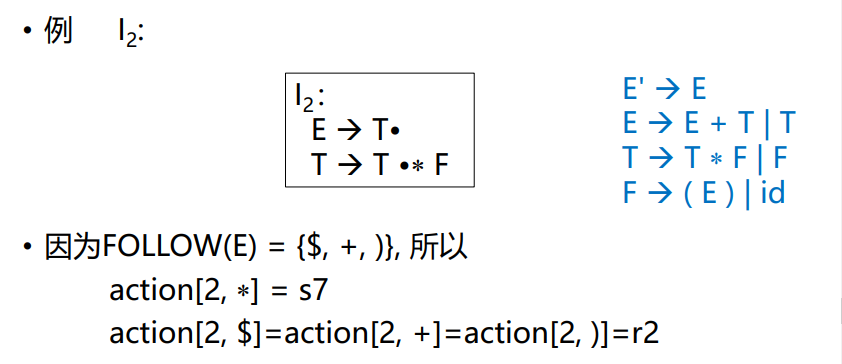文法特点：
SLR(1)文法都不是二义的，但是很多非二义的文法都不是SLR(1)的。
文法的描述能力有限，因为对于相同的字母，在不同情况下的归约方式可能会不同。
缺点解决方法：
加上后继搜索符，转变成LR（1）
LR（1）
将SLR的项目带上搜索符。部分基于SLR（1）会产生移进-规约冲突的例子，在基于LR（1）时没有冲突。如：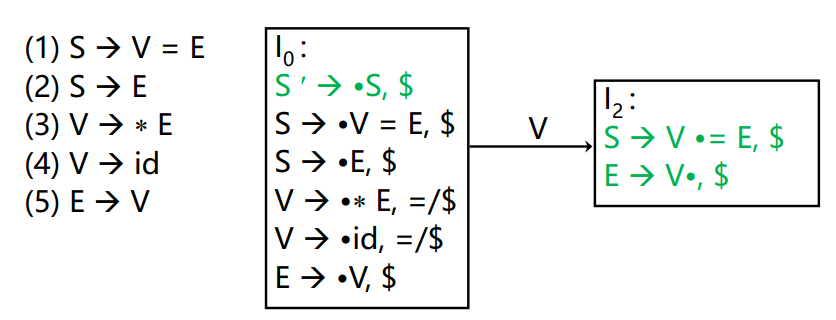缺点：
相当于是把SLR（1）中可能产生分歧的状态通过搜索符划分成了多个不会产生冲突的状态，因此状态数大大增加。
解决思路：
提出LALR分析表，功能介于SLR（1）和LR（1）之间，但是状态数与SLR（1）一样多。
LALR
构造方法：
通过合并规范LR（1）项目集得到；也就是说，通过损失一点点的精度来达到减少状态数量的目的。因为合并是有可能产生新的归约-归约冲突。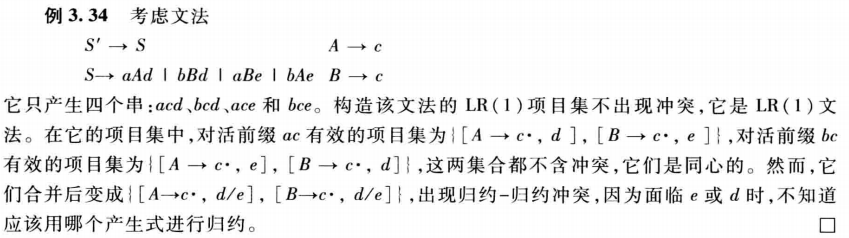总结：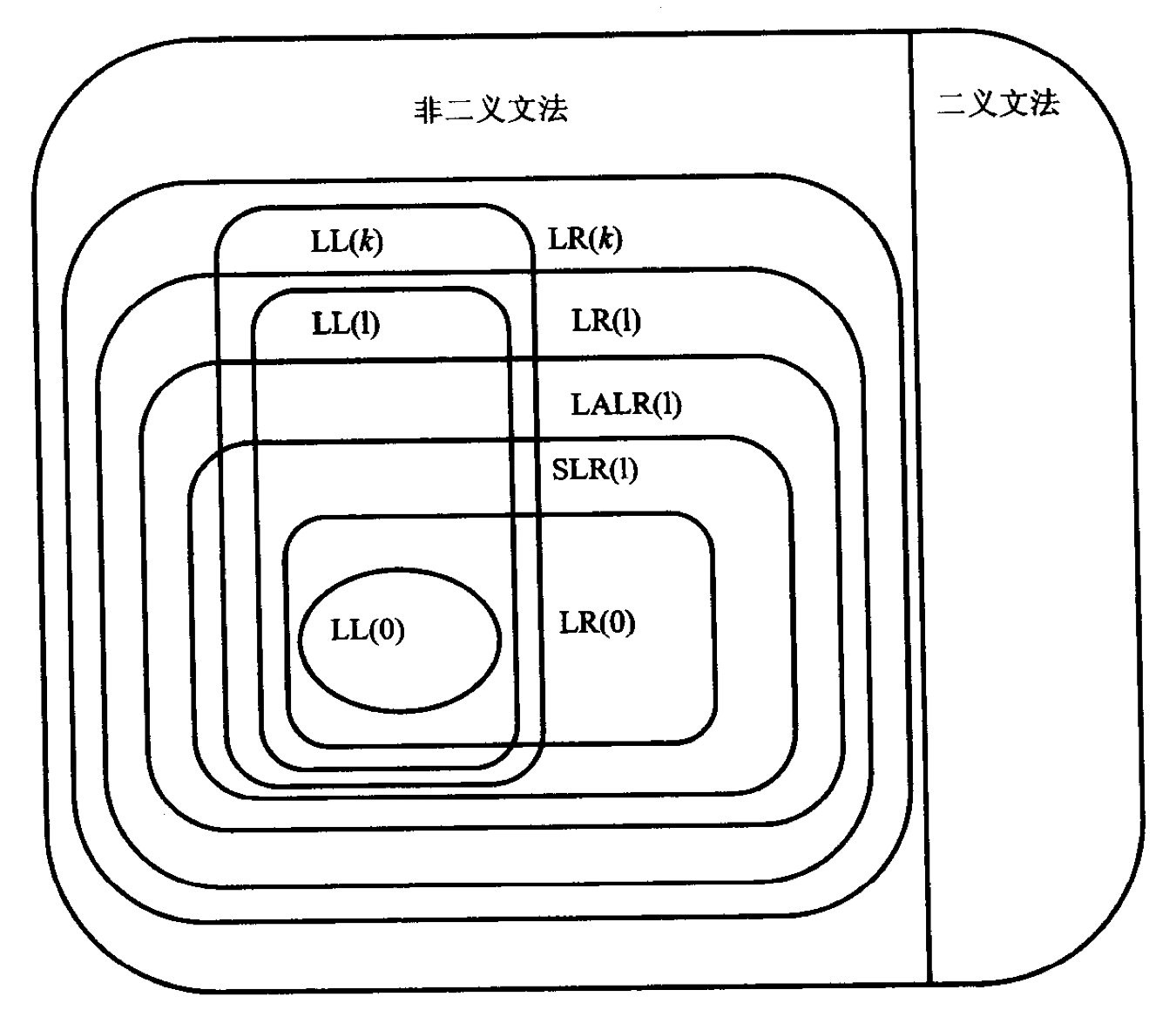附：LL（1）算法、LR（1）、算符优先方法三种分析方法比较：以上部分属于自己总结，部分截取自电子书与老师课件。
有什么问题欢迎大家指出。


展开全文• 是否是SLR(1)文法？？ 是否是LALR(1)文法？？ 是否是LR(1)文法？？ 依次说明理由。 解 FOLLOW集 (1)基于LR(0)项目的识别活前缀的DFA (2)基于LR(0)、LR(1)项目的识别活前缀的DFA 判断： 基于LR(0)项目的识别活...编译原理 学习笔记
• 是否是SLR(1)文法？？ 是否是LALR(1)文法？？ 是否是LR(1)文法？？ 依次说明理由。 解 （1）基于LR(0)项目识别活前缀的DFA、 暂无，参考上一篇博文。 （2）基于LR(1)项目识别活前缀的DFA 判断： 是LR(1) 的，因为...编译原理 学习笔记
• 编译Bottom-Up习题解答 精品文档 精品文档 收集于网络如有侵权请联系管理员删除 ...(2)构造识别该文法所产生的活前缀的DFA (3)构造其SLR分析表并判断文法是否是SLR(1)文法 解题思路 构造LR(0)项目集规范族有两种方
• 1 题 已知文法 AaAd|aAb| 判断文法是否是 SLR(1)文法若是构造相应分析表并对输入串 ab#给出分析过程 答案 文法 A aAd|aAb| 拓广文法为 G增加产生式 S A 若产生式排序为 0S' A 1 A aAd 2 A aAb 3 A 由产生式知 ...
• 1 题 已知文法 AaAd|aAb| 判断文法是否是 SLR(1)文法若是构造相应分析表并对输入串 ab#给出分 析过程 答案 文法 AaAd|aAb| 拓广文法为 G增加产生式 S A 若产生式排序为 0 S' A 1 A aAd 2 A aAb 3 A 由产生式知 ...
• 构造算符优先表二 、LR分析器LR（0）文法SLR1文法：LALR（1文法：LR（1文法： 一，短语，直接短语，句柄，素短语 定义 如果 β 中至少含有一个终结符，而且除它自身之外不再含任何更小的素短语，那么称 ...
• 判断是否为LL、LR、SLR文法（3.21，3.22，3.24） 构造LL（1）、LR（1）、SLR、LALR项目集及分析表 递归下降预测分析（函数形式） 移进、规约对应的缩写字母 si：移进，状态i进栈 rj：按产生式（j）规约 acc：表示...
• LR（0）文法判定、SLR文法判定及分析表、给定字符串写出分析过程 小题（自己复习时总结的） 计算机高级语言一般都有关键字、标识符、常数、运算符 和定界符这5类单词 基于生成观点、计算观点和识别观点，分别...
• 判断是否为SLR(1)文法的方法是什么？SLR(1)的特点是什么？ LR(0)文法要求文法的每一个LR(0)项目都不含有冲突的项目，这个条件比较苛刻。对于大多数程序设计语言来说，一般都不能满足LR(0)文法的条件。移进冲突规约...
• LL1文法判断 正规表达式求NFA 打开idea 输入正规表达式生成NFA与DFA 输入单词判断是否符合正规表达式 LL1文法，分析表 输入LL1文法 解析文法，消除左递归，提取公共左因子 计算First集合 计算Follow集合...
• 计算机网络技术重点知识结构 在计算机网络技术的学习、考试、应用中都有...5.判断文法是LR（0）还是SLR1），并构造其分析表？ 6.对于给定的属性文法和输入符号串α，该翻译方案的输出是什么？（需要给出解题过程）
• 构造SLR(1)分析程序，利用它进行语法分析，判断给出的符号串是否为该文法识别的句子，了解SLR1）分析方法是严格的从左向右扫描，和自底向上的语法分析方法。string fp 数据结构 action 算法
• 一、简答题(高频考点) 1.运行时的DISPLAY表的内容是什么？...判断、证明是（SLR1、LL1、LALR1）文法 构造预测分析表 输入串给出分析过程 4.算符优先分析 求FISTVT和LASTVT 构造算符优先分析表、判断是否为算
• 对于文法 ...1、算符优先分析法（需要先来判断文法是否为算符优先文法） 2、LR（0）分析法 3、SLR1）分析法 该程序的功能为，给定输入，程序按照先后顺序将使用的产生式输出。 如，输入25.6 *...